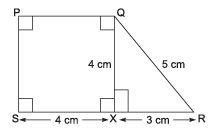# Very Short Answer Type Questions- Areas of Parallelograms and Triangles Notes | Study Mathematics (Maths) Class 9 - Class 9

## Class 9: Very Short Answer Type Questions- Areas of Parallelograms and Triangles Notes | Study Mathematics (Maths) Class 9 - Class 9

The document Very Short Answer Type Questions- Areas of Parallelograms and Triangles Notes | Study Mathematics (Maths) Class 9 - Class 9 is a part of the Class 9 Course Mathematics (Maths) Class 9.
All you need of Class 9 at this link: Class 9

Question 1. Find the area of trapezium PQRS given in the figure.Solution: Area of trapezium PQRS = ar (||gm PQXS) + ar (rt ΔQRX)
= (4 x 4)cm2 + (1/2) x 3 x 4) cm 2
= 16 cm2 + 6 cm2
= 22 cm2

Question 2. Adjacent sides of a rectangle are 16 cm and 8 cm. Find the area of the rectangle.
Solution:
Area of ractangle
= 16 cm x 8 cm = 128 cm

Question 3. A pair of parallel sides of a parallelogram measure 10 cm each. The perpendicular distance between them is 6 cm. What is the area of the parallelogram
Solution:
Area of a ||gm = Base x Altitude
= 10 cm x 6 cm = 60 cm2

The document Very Short Answer Type Questions- Areas of Parallelograms and Triangles Notes | Study Mathematics (Maths) Class 9 - Class 9 is a part of the Class 9 Course Mathematics (Maths) Class 9.
All you need of Class 9 at this link: Class 9Use Code STAYHOME200 and get INR 200 additional OFF

## Mathematics (Maths) Class 9

73 videos|351 docs|110 tests

Track your progress, build streaks, highlight & save important lessons and more!

,

,

,

,

,

,

,

,

,

,

,

,

,

,

,

,

,

,

,

,

,

;